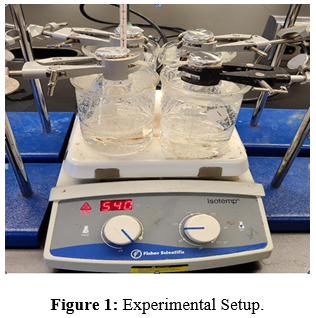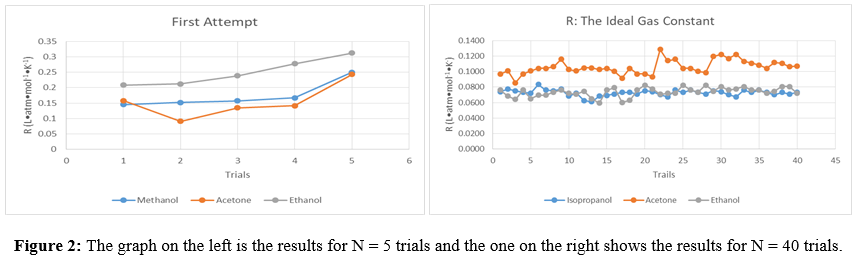## "Universal Gas Constant of Volatile Liquids"

### Moges Abebe1* and Bisrat Hailemeskel2

1Department of Biological and Physical Sciences, Saint Augustine University, USA

2Department of Clinical & Administrative Pharmacy Sciences, College of Pharmacy, Howard University, USA

*Corresponding author: Moges Abebe, Department of Biological and Physical Sciences, Saint Augustine University, USA

Received Date: 31 December, 2020; Accepted Date: 07 January, 2021; Published Date: 12 January, 2021

#### Abstract

Every year students at Saint Augustine University have an assignment to use the ideal gas equation, PV = nRT to determine the gram molecular weight of volatile liquids. This year they were asked to determine the universal gas constant experimentally and also give a hypothesis predicting whether the gas constant will be equal to or, more or less than the accepted value. After they performed the experiment they compared their results with their predictions. The null hypothesis is the accepted value of H0: R = 0.08206 L∙atm*mol-1*K-1 and the alternate hypothesis is what the students are proposing Hal: R < 0.082 L∙atm*mol-1*K-1 or HaR: R > 0.082 L∙atm*mol-1*K-1. Their results are presented as percentage errors and a statistical test was applied to the three volatile liquids: methanol, ethanol, and acetone. The results are presented in this study.

#### Introduction

Hypothesis testing can provide an excellent platform to teach students principles in chemistry while enabling handson application of the gas laws. In this paper twenty students, participated to determine the gas constant for three different volatile liquids using the ideal gas equation. The volatile liquids selected (methanol, ethanol, and acetone) had boiling points below 100°C. Prior to the laboratory experiment students were challenged to intuitively identify possible errors that could occur during the experiment and to answer pre-laboratory questions. Stating a hypothesis required them to prepare ahead, answer prelab questions and visualize the experiment before the lab began. Students were also encouraged to watch videos in order to visualize the laboratory procedure and appropriate use of equipment. This helped them to deepen their understanding of the Ideal Gas Law and develop critical and quantitative thinking skills. The students were told that the gas constant was R = 0.08206 L•atm•mol-1•K-1. Knowing the expected result reduced the anxiety students experienced as this was their first time performing the experiment. The extra preparation reduced the students misunderstanding and created a flowing procedural cadence during the experiment. Thus the hypothesis testing method is found to be a practical way to build creativity and develop unconventional problem-solving skills.

The step by step process for the hypothesis testing was as follows. Students were asked to state the null and alternate hypothesis (null hypothesis being H0 : μ = 0.08206 L•atm•mol-1 •K-1 while the alternate hypothesis was Ha: μ ≠ 0.08206 L•atm•mol-1•K-1) and were asked to include the reason for their choice. The students research and preparation before lab paid off in this step as they had already attained the necessary information to make a decision and develop their reasons. After performing the experiment the remaining step was to calculate the universal gas constant for each volatile liquid and be in a position to reject or fail to reject the null hypothesis. The null and alternate hypotheses are stated below:

H0: µ = 0.082 L∙atm*mol-1*K-1.

H1: µ > 0.082 L∙atm*mol-1*K-1

H2: µ < 0.082 L∙atm*mol-1*K-1.

#### Experimental Setup

The experimental setup is a standard 125mL Erlenmeyer flask covered with pin-holed aluminum foil and immersed in a 400 ml beaker water-bath and with the help of a heater the temperature of the water bath is raised to boiling. The mass of the condensed liquid, the atmospheric pressure, the volume of the Erlenmeyer flask, and the temperature of the boiling water were used to calculate the universal gas constant. The slope of PV/T for each gas is recorded.

#### Results

In the ideal gas equation R = PV/nT, P and V in the numerator are directly related to the R and any % error for each variables in the equation will result in the same % error on the gas constant. Care has to be given for the variables in the denominator, the temperature is in kelvin and the effects of the % error in temperature measurement have a slightly different effect than direct calculations. The mass of the condensed liquid is very small and any weight errors will be magnified when calculating the gas constant. Compared to the first trial with five samples, extra caution was taken for the 40 trials and the results showed major improvement. Students were asked to predict the R values before the experiment, whether the experimental results were going to be more or less than the ideal gas constant, R = 0.08206 L•atm•mol-1•K-1. Their predicted results for acetone was 40% correct, for ethanol 20%, and for methanol 80% accuracy. A better method of stating their hypothesis was needed (Table 1).

Table 2 shows the experimental results for two trials. The first set of five trials gave a mean value of 0.1745 for methanol, 0.1540 for acetone and 0.2499 for ethanol. The experimental results for 40 trials gave better values, 0.0727 for methanol, 0.1059 for acetone, and 0.0737 for ethanol.

The results for the two-tailed test for the volatile liquids is shown in Table 2. The 95% confidence test has two tails with the area below 0.025 and the results show that all the Z values are above that range.

#### Discussion

Even though STEM students are familiar with the importance of stating a good hypothesis as the foundation of their experiment, they have to be aware of two important things. The first is to be attentive that the guessing part when stating a hypothesis has to be reduced by answering the prelab questions, rewriting the procedure, and manipulating essential equations so that they are familiar with the variables in the equation. In this report, we have used the Ideal Gas Law equation for volatile liquids to determine R, the gas constant. The hypothesis was testable and the report goes further by testing the hypothesis to reject or fail-to-reject the null hypothesis. The null hypothesis H0: µ = 0.082 L∙atm*mol-1*K-1 has been accepted for many decades as the true value for the ideal gas law. For real gases, the ideal gas law is known to deviate by 5% at a standard temperature and pressure and thus the alternate hypothesis was needed: Ha: µ ≠ 0.082 L∙atm*mol-1*K-1.

To test the null hypothesis the result of five samples of the volatile liquids were graphed in figure 2. From the five trials for the volatile liquids the mean values for methanol (0.1745), ethanol (0.1540), and acetone (0.2499) showed a major deviation from the R = 0.082 L∙atm*mol-1*K-1. Further investigation using 40 samples of isopropyl alcohol (0.0727), acetone (0.1059) and ethanol (0.0737) gave better results but the error was still above 10%. Previous work1 has stated that the Van der Waals equation was applied to calculate the molecular weight of volatile liquids; the results showed major improvement. Results from 40 samples and the use of standard deviation of a two-tailed z testshowed that there was enough evidence to reject the null hypothesis. Furthermore, a P test was conducted and the sufficiency of the evidence validated the decision to reject the null hypothesis.

#### Conclusion

This paper shows how the use of the familiar ‘educated guess’ definition of the term hypothesis is used to reduce guesswork and better equip students to improve estimated outcomes. Both preparation and familiarity with experiment protocols help students announce testable hypothesis with accuracy that had a measurably higher accuracy than by-chance predictions. We changed our strategy and offered a limited choice of hypothetical statements as more-or-less than the Universal constant; the student understanding and confidence increased noticeably. The introduction part of the lab protocol had historical development, sample calculations, and a summary of the experimental procedure. From this information and the introductory lecture, students had enough information to ask the research question and state a good hypothesis. In the gas constant determination of these experiments emphasis was given to the source of error and students were efficient, avoided most of the errors, and found the accurate mass of the condensed liquid.

The result from testing the hypothesis shed a different light on the universal gas constant for volatile liquids. The two-tailed z test on the 40 samples gave enough information to reject the null hypothesis for the three volatile liquids in this experiment. The P test also confirmed rejection of the null hypothesis on the universal gas constant. Rejecting the Universal Gas Constant for ideal gases, R = 0.082 L∙atm*mol-1*K-1 opens up possibilities for future research of volatile liquids which have boiling points below 100°C. The deviation from the universal gas constant reduces the stringent requirement by educators on the student for the near-zero percentage error and reasonable standard deviation so that the focus will be more on the explanation of the results more than the accuracy of the result. We suggest that educators require the necessary groundwork needed by each student that will enable them to state a strong and testable hypothesis and try to avoid unnecessary human error. This opens up opportunities for educators to accumulate results from the entire class and to further challenge accepted laws and theories.

Acknowledgment to the students at Saint Augustine University that participated in this experiment: Early college students: Bista, Garima; Coleman, Sierra; Foust, Samantha; Hughes, Spencer; Ingram, Judaea; Osman, Kinsie. Traditional university students: Bain, Richara; Barco, Zachary; Bishop, Landon Daront’e; Bowe, Bianca Rickia; Coppedge, Maleak Javon; Eggleston, Brashaunta; Gilmore, Quiera Kamille; Hammond, Angela Antris; Hart, Kennitha M.; Hawkins, Rodney Montel; Key, Breauna Janea; McNeill, James Terrence; Wright, Darriel.N = 5 Trials N = 40 Trials Methanol Acetone Ethanol Isopropanol Acetone Ethanol Mean 0.1745 0.1540 0.2499 0.0727 0.1059 0.0737 Standard D 0.04276 0.0557 0.04462 0.00398 0.00891 0.0059 t score 9.63087 5.74752 16.7857 -10.9363 11.7907 -6.539 p-values 0.00065 0.004543 0.000074 0.06951 0.06951 0.0695

Table 1: Experimental results for two sets of trial where N = 5 and N = 40.

 Volatile liquids H0:μ = Ha: μ≠ X̅ s n X̅ -μ0 (s/√n) Z test Isopropyl alcohol 0.08206 ≠ 0.08206 0.0727 0.003981 40 -0.0094 0.000629 -14.9261 Acetone 0.08206 ≠ 0.08206 0.1059 0.008913 40 0.0238 0.001409 16.91585 Ethanol 0.08206 ≠ 0.08206 0.0737 0.005937 40 -0.0083 0.000939 -8.88596

Table 2: The calculated results for the Z test.

Citation: Abebe M, Hailemeskel B (2021) Universal Gas Constant of Volatile Liquids. Arch Nat Med Chem 6: 125. DOI: 10.29011/2577-0195.000125